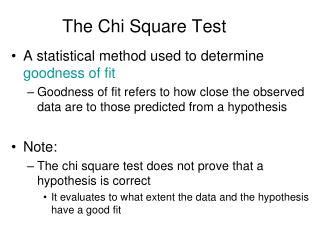DownloadDownload PresentationThe Chi Square Test

# The Chi Square Test

Download Presentation## The Chi Square Test

- - - - - - - - - - - - - - - - - - - - - - - - - - - E N D - - - - - - - - - - - - - - - - - - - - - - - - - - -
##### Presentation Transcript

1. The Chi Square Test • A statistical method used to determine goodness of fit • Goodness of fit refers to how close the observed data are to those predicted from a hypothesis • Note: • The chi square test does not prove that a hypothesis is correct • It evaluates to what extent the data and the hypothesis have a good fit

2. The Chi Square Test (we will cover this in lab; the following slides will be useful to review after that lab) • The general formula is (O – E)2 c2 =S E • where • O = observed data in each category • E = observed data in each category based on the experimenter’s hypothesis • S = Sum of the calculations for each category

3. Gene affecting wing shape • c+ = Normal wing • c = Curved wing • Gene affecting body color • e+ = Normal (gray) • e = ebony • Consider the following example in Drosophila melanogaster • Note: • The wild-type allele is designated with a + sign • Recessive mutant alleles are designated with lowercase letters • The Cross: • A cross is made between two true-breeding flies (c+c+e+e+ and ccee). The flies of the F1 generation are then allowed to mate with each other to produce an F2 generation.

4. The outcome • F1 generation • All offspring have straight wings and gray bodies • F2 generation • 193 straight wings, gray bodies • 69 straight wings, ebony bodies • 64 curved wings, gray bodies • 26 curved wings, ebony bodies • 352 total flies • Applying the chi square test • Step 1: Propose a null hypothesis (Ho) that allows us to calculate the expected values based on Mendel’s laws • The two traits are independently assorting

5. Step 2: Calculate the expected values of the four phenotypes, based on the hypothesis • According to our hypothesis, there should be a 9:3:3:1 ratio on the F2 generation

6. (O1 – E1)2 (O2 – E2)2 (O3 – E3)2 (O4 – E4)2 E1 E2 E3 E4 (193 – 198)2 (69 – 66)2 (64 – 66)2 (26 – 22)2 66 66 22 198 • Step 3: Apply the chi square formula c2 = + + + c2 = + + + c2 = 0.13 + 0.14 + 0.06 + 0.73 c2 = 1.06

7. Step 4: Interpret the chi square value • The calculated chi square value can be used to obtain probabilities, or P values, from a chi square table • These probabilities allow us to determine the likelihood that the observed deviations are due to random chance alone • Low chi square values indicate a high probability that the observed deviations could be due to random chance alone • High chi square values indicate a low probability that the observed deviations are due to random chance alone • If the chi square value results in a probability that is less than 0.05 (ie: less than 5%) it is considered statistically significant • The hypothesis is rejected

8. Step 4: Interpret the chi square value • Before we can use the chi square table, we have to determine the degrees of freedom (df) • The df is a measure of the number of categories that are independent of each other • If you know the 3 of the 4 categories you can deduce the 4th (total number of progeny – categories 1-3) • df = n – 1 • where n = total number of categories • In our experiment, there are four phenotypes/categories • Therefore, df = 4 – 1 = 3 • Refer to Table 2.1

9. 1.06

10. Step 4: Interpret the chi square value • With df = 3, the chi square value of 1.06 is slightly greater than 1.005 (which corresponds to P-value = 0.80) • P-value = 0.80 means that Chi-square values equal to or greater than 1.005 are expected to occur 80% of the time due to random chance alone; that is, when the null hypothesis is true. • Therefore, it is quite probable that the deviations between the observed and expected values in this experiment can be explained by random sampling error and the null hypothesis is not rejected. What was the null hypothesis?